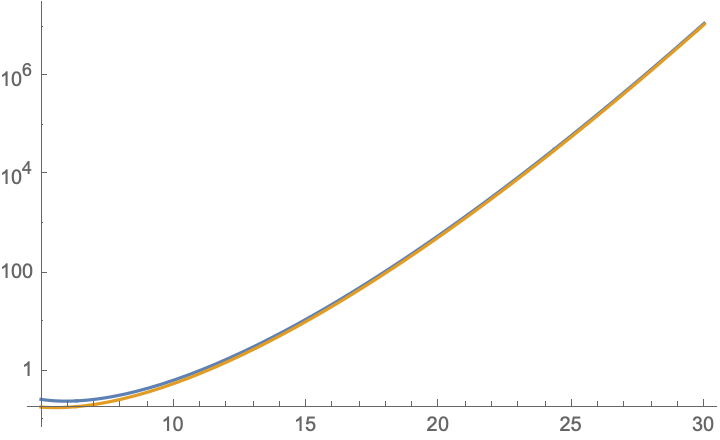# Integral rising from difference of chi-squared random variables

Let $$X,Y$$ be independent random variables such that $$X\sim\chi_{n-1}^{2}, Y\sim\chi_{1}^{2}$$ are chi-squared distributed (where $$n\geq2$$ is a natural number). I am trying to evaluate $$\mathbb{P}[X\leq Y]$$ as a function of $$n$$, at least asymptotically when $$n\to\infty$$. Obviously this probability decays to 0, but I'd like to be able to say something more quantative.

I was able to reduce this into a purely analytic question: Denoting $$Z=X-Y$$, I'm seeking for $$F_Z(0)$$. Expressing $$F_Z$$ using $$f_X,F_Y$$ I found that $$\mathbb{P}[X\leq Y]=\frac{1}{2^{\frac{n-1}{2}}\Gamma(\frac{n-1}{2})}\int_{0}^{\infty}\left(erfc(\sqrt{x})x^{\frac{n-3}{2}}e^{-\frac{x}{2}}\right)dx$$ where $$erfc$$ is the complementary error function.

To my suprise, wolfram spits out nice looking algebraic numbers for odd $$n$$, which gives me hope that this integral can be expressed as a (simpler) function of $$n$$. Any help, either evaluating the integral or calculating the asymptotic probability in some other way will be much appreciated.

$$F_n=\int_{0}^{\infty}{\rm erfc}\,(\sqrt{x})x^{\frac{n-3}{2}}e^{-\frac{x}{2}}dx$$ $$\qquad\qquad=2^{2-n} \, _2F_1\left(\tfrac{n-1}{2},\tfrac{n}{2};\tfrac{n+1}{2};-\tfrac{1}{2}\right)\frac{\Gamma \left(n-1\right)}{\Gamma \left(\frac{n}{2}+\frac{1}{2}\right)}.$$ The large-$$n$$ asymptotics follows by approximating $${\rm erfc}(\sqrt{x})\simeq \frac{e^{-x}}{\sqrt{\pi x } }$$, resulting in $$F_n\rightarrow\pi^{-1/2}\left(\tfrac{3}{2}\right)^{1-\frac{n}{2}} \Gamma \left(\tfrac{n}{2}-1\right),\;\;n\gg 1.$$ This plot compares $$F_n$$ (gold) and the large-$$n$$ asymptotic (blue), they are nearly indistinguishable for $$n$$ above 10 or so.For the probability in the OP I thus find for $$n\gg 1$$ $$\mathbb{P}[X\leq Y]\rightarrow \frac{3^{1-\frac{n}{2}} \Gamma \left(\frac{n}{2}-1\right)}{\sqrt{2 \pi } \Gamma \left(\frac{n-1}{2}\right)},\;\;n\gg 1.$$

• Looking at asymptotics for $\Gamma$, you could also say $$\mathbb{P}[X\leq Y]\rightarrow \sqrt{\frac{3^{2-n}}{\pi n}},\;\;n\gg 1.$$
– user44143
Apr 21, 2020 at 13:14
• @MattF. --- certainly, thanks. Apr 21, 2020 at 13:19
• Thanks! You wrote that $erf(\sqrt{x})\simeq e^{-x}/\sqrt{\pi x}$, isn't it supposed to be $erf(\sqrt{x})\simeq 1-e^{-x}/\sqrt{\pi x}$?
– GuyK
Apr 21, 2020 at 13:49
• Also, are you willing to elaborate on the first equality? I do not see how the hypergeometric function pops up.
– GuyK
Apr 21, 2020 at 13:56
• @GuyK -- indeed, I wrote erf when I meant erc (as in your integral); the first equality is simply Mathematica output; but in any case the asymptotics is already so accurate for moderate $n$, that you will probably not need the hypergeometric function. Apr 21, 2020 at 14:19